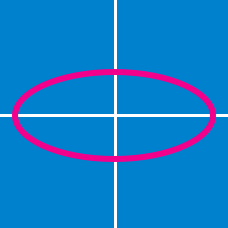Geometry

# Conics - Circle Standard Equation

What is the equation of the circle passing through the points $$(0,0)$$ and $$(6,12)$$ with center on the line $$y=x+3?$$

What is the equation of the circle which passes through the two points $$(-3,0)$$ and $$(7,4)$$ and is centered at a point on the $$y$$-axis?

If $$(-2, -6)$$ and $$(8, 8)$$ are two endpoints of a diameter of a circle, what is the equation of the circle?

What is the equation of the circle tangent to the $$x$$-axis with center $$(5,-1)$$?

If the circle $x^2+y^2-8x+2y +1=0$

has center $$(a,b)$$ and radius $$r,$$ what is the value of $$a+b+r ?$$

×